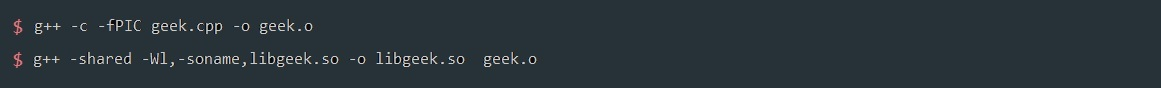Related Articles

# How to call C / C++ from Python?

• Last Updated : 29 Jul, 2020

In order to take advantage of the strength of both languages, developers use Python bindings which allows them to call C/C++ libraries from python.

Now, the question arises that why there is a need for doing this?

1. As we know that, C has faster execution speed and to overcome the limitation of Global Interpreter Lock(GIL) in python, python bindings are helpful.
2. We have a large, stable and tested library in C/C++, which will be advantageous to use.
3. For performing large scale testing of the systems using Python test tools.

Let’s see the C code which we want to execute with Python :

## C++

 `#include ``class` `Geek{``    ``public``:``        ``void` `myFunction(){``            ``std::cout << ``"Hello Geek!!!"` `<< std::endl;``        ``}``};``int` `main()``{``    ``// Creating an object``    ``Geek t; `` ` `    ``// Calling function``    ``t.myFunction();  ``  ` `    ``return` `0;``}`

We have to provide those cpp declarations as extern “C” because ctypes can only interact with C functions.

## C++

 `extern` `"C"` `{``    ``Geek* Geek_new(){ ``return` `new` `Geek(); }``    ``void` `Geek_myFunction(Geek* geek){ geek -> myFunction(); }``}`

Now, compile this code to the shared library :Finally, write the python wrapper:

## Python3

 `# import the module``from` `ctypes ``import` `cdll`` ` `# load the library``lib ``=` `cdll.LoadLibrary(``'./libgeek.so'``)`` ` `# create a Geek class``class` `Geek(``object``):`` ` `    ``# constructor``    ``def` `__init__(``self``):`` ` `        ``# attribute``        ``self``.obj ``=` `lib.Geek_new()`` ` `    ``# define method``    ``def` `myFunction(``self``):``        ``lib.Geek_myFunction(``self``.obj)`` ` `# create a Geek class object``f ``=` `Geek()`` ` `# object method calling``f.myFunction()`

Output :

```Hello Geek!!!
```

Attention geek! Strengthen your foundations with the Python Programming Foundation Course and learn the basics.

To begin with, your interview preparations Enhance your Data Structures concepts with the Python DS Course. And to begin with your Machine Learning Journey, join the Machine Learning – Basic Level Course

My Personal Notes arrow_drop_up